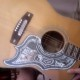### duality in the 2-dimensional projective space over the field with 4 elements

new drawing today.

you can check my work…
as of course i already *have*:
verify on five cross-diagrams
(by shading one “circle” on each…
namely the one whose position
corresponds to that of C
in the “big picture”) that
the “Point-at-C” belongs
to each of the “Lines-through-C”.

i’ll edit in some algebra; probably soon.
but the point now is that i *don’t* need
to refer to any but purely *visual*
calculations.

somewhat to my surprise, i’ve decided
in middle age to become much more
of a “visual learner”. in my case, this
amounts to “how can i represent results
from abstract algebra as pictures?”.
it still counts.

1.vlorbik

the real title is${P^2({\Bbb F}_4)}^*$.

2.vlorbik${\Bbb F}_4 = \{0, 1, a, b\}$
as a set. its addition and multiplication
tables are

+01ab
001ab
110ba
aab01
bba10

*01ab
00000
101ab
a0ab1
b0b1a
.

consider$F_4 \times F_4$
(ordered pairs [x,y] with
x, y \in {0,1,a,b}…). tack on
a “z co-ordinate” of 1 to each point
to obtain the so-called homogenous co-ordinates
for the “finite points” of P_2(F_4):

(a,a,1) (a,b,1) | (b,a,1) (b,b,1)
(a,0,1) (a,1,1) | (b,0,1) (b,1,1)
————————————-
(0,a,1) (0,b,1) | (1,a,1) (1,b,1)
(0,0,1) (0,1,1) | (1,0,1) (1,1,1)

the other five points… forming
“the line at infinity” are then
(1,0,0) [the center in my drawing]
(0,1,0) [the bottom]
(a,1,0) [left-hand]
(b,1,0) [right-hand] and
(1,1,0) [top].

“dualizing” can now be expressed by
considering *lines* having co-ordinates
[X,Y,Z].

a point (p,q,r) is then “on the line” [X,Y,Z]
if and only if Xp + Yq + Zr = 0
(as an element of F_4 [of course!]).

a^2 = b
b^2 = a
a+b+1 =0
ab = ba = 1
and suchlike equations come into play
over and over in calculating out the drawing.
after a while F_4 gets pretty familiar.

• ## (Partial) Contents Page

Vlorbik On Math Ed ('07—'09)
(a good place to start!)